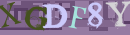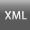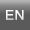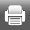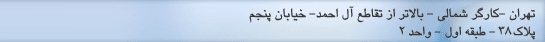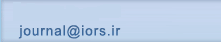[صفحه اصلی ]   [Archive]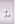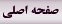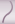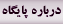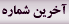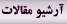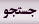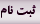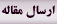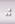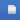جلد 9، شماره 1 - ( 4-1397 )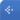جلد 9 شماره 1 صفحات 1-28 برگشت به فهرست نسخه ها
A goal programming approach for fuzzy flexible linear programming problems
چکیده:   (503 مشاهده)
We are concerned with solving Fuzzy Flexible Linear Programming (FFLP) problems. Even though, this model is very practical and is useful for many applications, but there are only a few methods for its situation. In most approaches proposed in the literature, the solution process needs at least, two phases where each phase needs to solve a linear programming problem. Here, we propose a method to solve the given problem in just one phase using only one problem. Furthermore, using our approach, sensitivity analysis of Fuzzy Flexible Linear Programming (FFLP) problem is simpler. For an illustration of our method, some numerical examples given. In particular, a practical problem is formulated and is solved by our method and several other methods and the obtained results are compared.
متن کامل [PDF 1047 kb]   (425 دریافت)
نوع مطالعه: پژوهشی | موضوع مقاله: Mathematical Modeling and Applications of OR
دریافت: 1396/8/19 | پذیرش: 1397/6/13 | انتشار: 1397/9/18
ارسال پیام به نویسنده مسئول

ارسال نظر درباره این مقاله
نام کاربری یا پست الکترونیک شما: